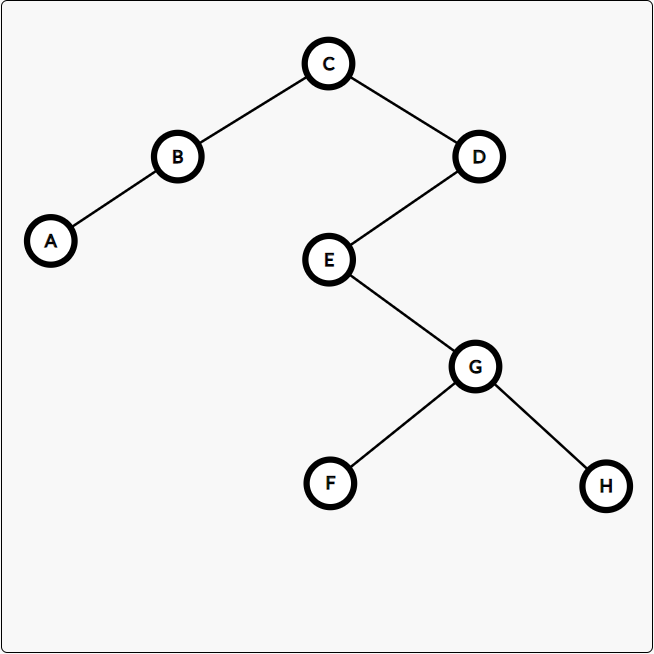CSP/S-2019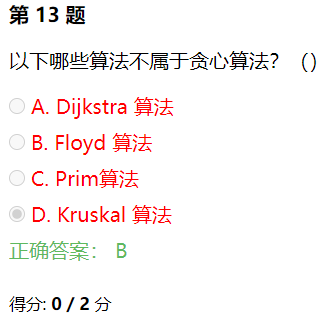​ Dijkstra 需要选取最近的 d[s]，Floyd 不需要任何贪心，Prim 同 Dijkstra，Kruskal 需要选取最短的边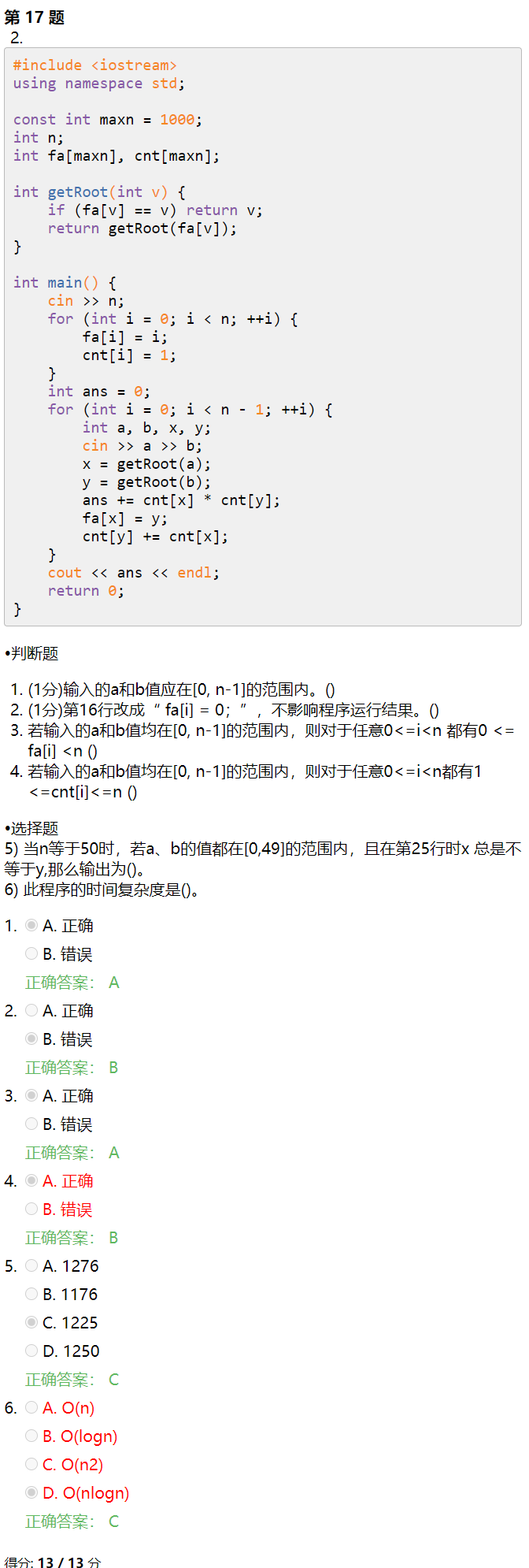模拟题自测

​ 显然选 C，因为很容易举出反例。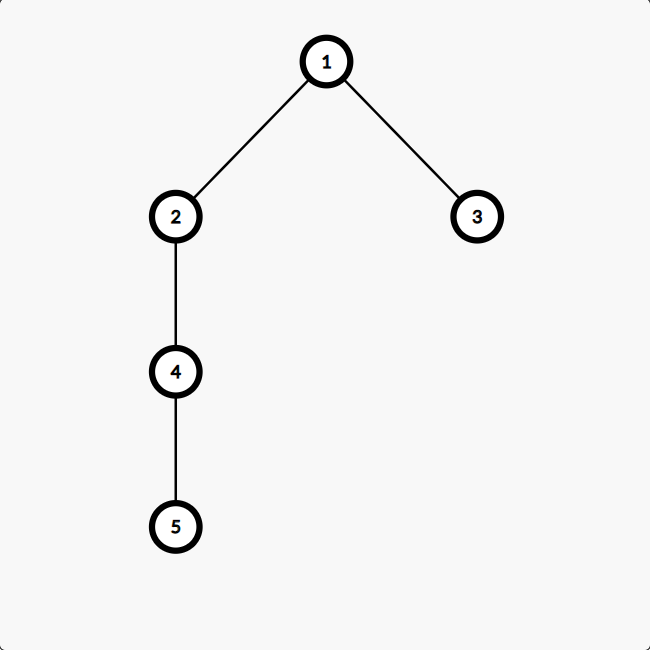memset(a,255,sizeof(a)) 等价于将 a 全部赋值为 $-1$

一些知识点总结

局域网、城域网、广域网、个人局域网

• LAN：局域网
• MAN：城域网
• WAN：广域网
• PAN：个人局域网

RAM 中的信息是 计算机工作时随机写入的（NOIP2000 普及）

• 1B=8bit
• 1KB=1024B
• 1MB=1024KB
• 1GB=1024MB
• 1TB=1024GB

二叉树的若干性质

• 在二叉树的第 $i$ 层上最多有 $2^{i-1}$ 个结点（$i \ge 1$）
• 深度为 $k$ 的二叉树至多有 $2^{k} - 1$ 个结点（满二叉树）
• 对于任意一棵二叉树，如果其叶结点数为 $n_0$，而度数为 $2$ 的结点总数为 $n_2$，则有 $n_0=n_2+1$
• 具有 $n$ 个结点的完全二叉树的深度为 $\lfloor \log_2n \rfloor+1$

遍历二叉树

知前序、中序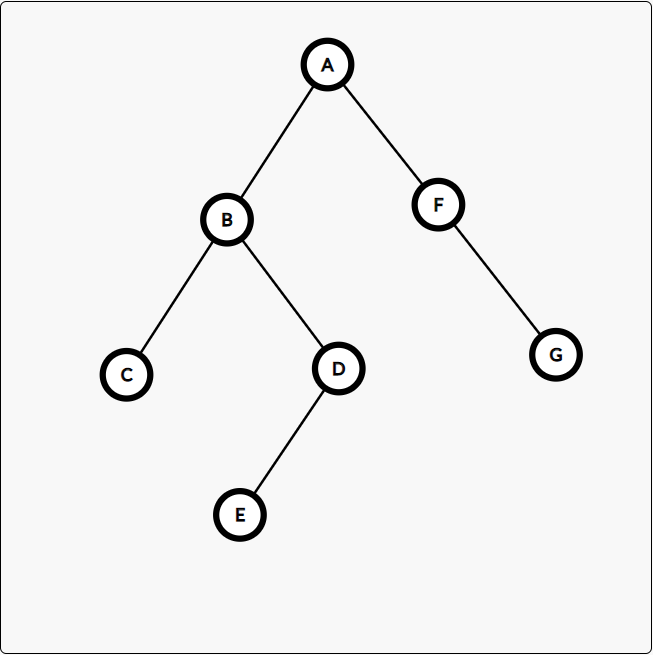知后序、中序